Home | Study Links | Poems |

## Alpha ( α )

For your consideration, proof the fine structure constant can be mathematically derived.

The fine-structure https://physics.nist.gov/cgi-bin/cuu/Value?alph|search_for=fine+structure
scientifically measured has an approximate value of 0.007297352569. Mostly recognized but not
the next digit, so I split the next digit possibilities 0-9 (5), added it, and began work from there.

1 / 0.007297352569(5) = 137.035999079940028 2 = 18778.865043837324229

My efforts to solve this mathematically began with 18977.06203214114801425... (π8 x 2).
It is very near to the above-mentioned number, and along with this starting number an end
number was required, like a book cover. (All numbers are entirely pi (π) and phi (ɸ) based.)

3.141592653589793238462 = 1 / 0.318309886183790671537 (1 / π)
3.141592653589793238462 x 2 = 6.283185307179586476925
0.020531964509368670378    /    6.283185307179586476925 =
0.003267763643053385472

9.869604401089358618834 = 1 / 0.101321183642337771443 (1 / π2)
9.869604401089358618834 x 2 = 19.73920880217871723766
0.020531964509368670378    /    19.73920880217871723766 =
0.001040161473295852296

97.40909103400243723644 = 1 / 0.010265982254684335189 (1 / π4)
97.40909103400243723644 x 2 = 194.8181820680048744728
0.020531964509368670378    /    194.8181820680048744728 =
0.000105390391653493666 = 1 / 9488.531016070574007128

1 -------------------------------   X   ------------------------------ (Base line and reversal point)

9488.531016070574007128 = 1 / 0.000105390391653493666 (1 / π8)
9488.531016070574007128 x 2 = 18977.06203214114801425 (π8 x 2)
0.020531964509368670378    /    18977.06203214114801425 =
0.0000010819358905289

90032220.84293327956713 = 1 / 0.000000011107134652876 (1 / π16)
90032220.84293327956713 x 2 = 180064441.6858665591342
0.020531964509368670378    /    180064441.6858665591342 =
0.000000000114025647246
Etc.

(2 / π4)                                  (π8 x 2)                                   (2 / π4) / (π8 x 2)
0.020531964509368670378… / 18977.06203214114801425… = 0.0000010819358905289

Divide this number by π4 (97.409091034002437236...) and continue:

0.0000010819358905289 / 97.409091034002437236 =
0.0000000111071346528 / 97.409091034002437236 =
0.0000000001140256472 / 97.409091034002437236 =
Etc.
Adding up all of the layers to infinity = 0.0000010931582335562 =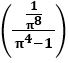A visual explanation: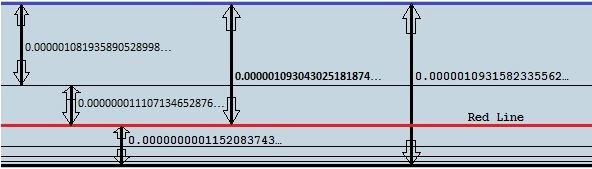(Perhaps these lines represent spectral lines)

0.020531964509368670378… / 18977.06203214114801425… = 0.0000010819358905289
0.020531964509368670378… / 18784.22352674753383243… = 0.0000010930430251818
0.020531964509368670378… / 18782.24385007314313978… = 0.0000010931582335562…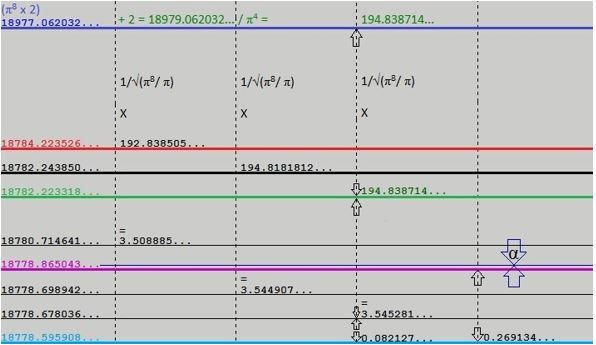18977.06203214114801425 - 192.83850539361418182 =
18784.22352674753383243 - 001.97967667439069265… =
18782.24385007314313978… - 000.02053196450936867… =
18782.22331810863377111

Dividing by π4:

192.83850539361418182… / 97.409091034002437236 =
...1.97967667439069265… / 97.409091034002437236 =
Etc.
...0.02053196450936867…

194.8387140325142431432… (π4 / .5) + (2 / π4)

Next step acquiring the end (bottom) number:

194.8387140325142431432/ √(π8/ π) =
3.545281301022101405403… +
0.082127858037474681513… (8 / π4) =
3.627409159059576086916…

18782.22331810863377 - 3.627409159059576086916… = 18778.59590894957419502…

18977.06203214114801425… (Start #) -
18778.59590894957419502 (End #) =
198.4661231915738192301… -
0.269134858448657890432… (explanation follows) = 198.1969883331251613…

18977.06203214114801425 - 198.1969883331251613… = 18778.86504380802285291
or
18778.59590894957419502+ 0.269134858448657890… = 18778.86504380802285291

While working on possibilities a major clue presented itself:
When I subtracted the following two numbers,

18778.865043837324229 (original number from the top of the page) -
18778.59590894957419502… (end #) =
0.26913488775003398… -
0.020531964509368670378… (2 / π4) =
0.248602923240665309622… 1/x =
4.022478846847383539716…2 =
16.18033607333465644263…

and noticing phi numbers, I reasonably deduced that phi is part of the solution!
I decided to run with it and reverse the process starting with (√1.25 + .5) x 10 =

16.18033988749894848204… √ =
4.022479320953551960229… 1/x =
0.248602893939289220053… +
0.020531964509368670378… (2 / π4) =
0.269134858448657890432+
18778.59590894957419502=
18778.86504380802285291… (original number now adjusted, and locked in by ɸ and π) =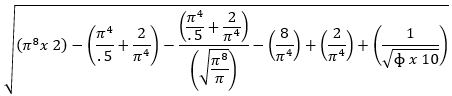137.035999079833116867867985771960524751261126710956764394872128728819663336
1/x
0.0072973525695056931682062262973500731680835106878962634963700448348384385

Perhaps this represents the limit of physical measurements. Phi is not included in the next and
final section (see below), which is entirely pi based and can only be attained mathematically.

I do not believe that these two special numbers 3168 and 31680 are random numbers, especially
so close together, but were placed deliberately. If so, perhaps all those numbers are a language!
Wouldn't it be amazing if it says, "In the beginning God created the heaven and the earth." ?

Three interesting facts:

1. The value for the name “Lord Jesus Christ” in Greek numeric is 3168, the number of his name.

2. Earths dimensions fitted into a square has a perimeter of 31680 miles (7920 x 4).

3. He was born southeast of Bethlehem, at latitude 31.68 degrees North.

Inserting the final section to complete the formula: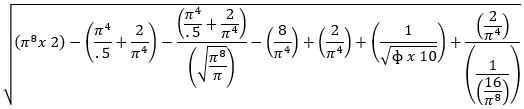137.035999206157399222318479001000262689537834609473995213691525285349733120
1/x
0.00729735256277875402980213640259738753519520968347474978298633008561682393

Observe the interesting number pattern in that last formula section: 1, 2, 4, 8, 16The first 3 sections span the distance between (π8 x 2) and the bottom line or end number.
From there the last 3 sections bounce back up to the alpha line, like a fold as in a curtain!

Time will tell, but I feel confident that my results are correct for two main reasons:
1. Phi is part of the solution, and 2. the appearance of numbers 3168 and 31680.
Can't wait to compare with the latest scientifically measured alpha digit(s).

Speed of light in miles per second:

18778.8650438 -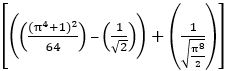= 18628.2396760… x 10 = 186282.396760…

18778.8650784-= 18628.2397106… x 10 = 186282.397106…